# 3.8 Plotting data files

In the simple example of the previous section, we plotted the first column of a data file against the second. It is possible to plot any arbitrary column of a data file against any other; the syntax for doing this is:

```plot 'data.dat' using 3:5
```

This example would plot the contents of the fifth column of the file data.dat on the vertical axis, against the contents of the third column on the horizontal axis. As mentioned above, columns in data files can be separated by whitespace and/or commas. Algebraic expressions may also be used in place of column numbers, as in the example:

```plot 'data.dat' using (3+\$1+\$2):(2+\$3)
```

In such expressions, column numbers are prefixed by dollar signs to distinguish them from numerical constants. The example above would plot the sum of the values in the first two columns of the data file, plus three, on the horizontal axis, against two plus the value in the third column on the vertical axis. The column numbers in such expressions can also be replaced by algebraic expressions, and so \$2 can also be written as \$(2) or \$(1+1). In the following example, the data points are all placed on the vertical line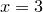– the brackets around the 3 distinguish it as a numerical constant rather than a column number – meanwhile their vertical positions are drawn from the value of some column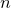in the data file, where the value ofis itself read from the second column of the data file:

```plot 'data.dat' using (3):\$(\$2)
```

It is also possible to plot data from only selected lines within a data file. When Pyxplot reads a data file, it looks for any blank lines in the file. It divides the data file up into data blocks, each being separated from the next by a single blank line. The first data block is numbered 0, the next 1, and so on.

When two or more blank lines are found together, the data file is divided up into index blocks. The first index block is numbered 0, the next 1, and so on. Each index block may be made up of a series of data blocks. To clarify this, a labelled example data file is shown in Figure 3.1.

 0.0  0.0 Start of index 0, data block 0. 1.0  1.0 2.0  2.0 3.0  3.0 A single blank line marks the start of a new data block. 0.0  5.0 Start of index 0, data block 1. 1.0  4.0 2.0  2.0 A double blank line marks the start of a new index. ... 0.0  1.0 Start of index 1, data block 0. 1.0  1.0 A single blank line marks the start of a new data block. 0.0  5.0 Start of index 1, data block 1.etc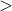Figure 3.1: An example Pyxplot data file – the data file is shown in the two left-hand columns, and commands are shown to the right.

By default, when a data file is plotted, all data blocks in all index blocks are plotted. To plot only the data from one index block, the following syntax may be used:

```plot 'data.dat' index 1
```

To achieve the default behaviour of plotting all index blocks, the index modifier should be followed by a negative number.

It is also possible to specify which lines and/or data blocks to plot from within each index. To do so, the every modifier is used, which takes up to six values, separated by colons:

```plot 'data.dat' every a:b:c:d:e:f
```

The values have the following meanings: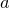Plot data only from everyth line in data file.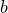Plot only data from everyth block within each index block.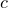Plot only from lineonwards within each block.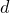Plot only data from blockonwards within each index block.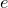Plot only up to theth line within each block.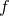Plot only up to theth block within each index block.

Any or all of these values can be omitted, and so the following are both valid statements:

```plot 'data.dat' index 1 every 2:3
plot 'data.dat' index 1 every ::3
```

The first would plot only every other data point from every third data block. The second would plot data from the third line onwards within every data block.

Comment lines may be included in data files by prefixing them with a hash character. Such lines are completely ignored by Pyxplot and do not count towards the one or two blank lines required to separate blocks and index blocks. It is often good practice to include comment lines at the top of data files to indicate their date and source. In Section 5.4 we will see that Pyxplot can read metadata from some comment lines which follow a particular syntax.